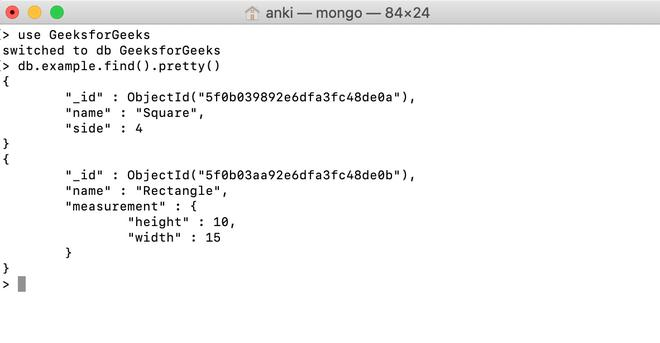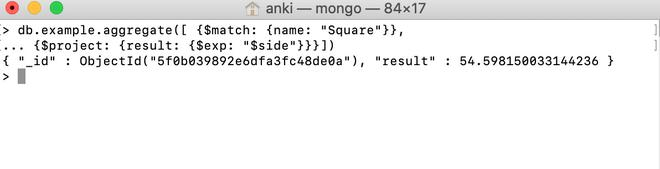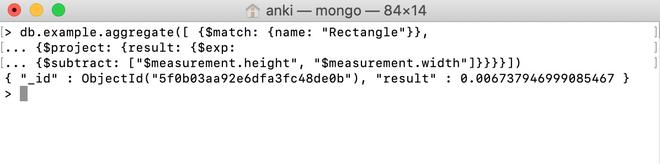# MongoDB \$exp Operator

MongoDB provides different types of arithmetic expression operators that are used in the aggregation pipeline stages \$exp operator is one of them. This operator is used to raise Euler’s number (i.e. e ) to the specified exponent and returns the result.

Syntax:

```{ \$exp: <exponent> }

```

Here, the exponent is a valid expression until it resolves to a number.

• If the entered value resolves to null, then this operator will return null.
• If the entered value resolves to NaN, then this operator will return NaN.
• If the entered value refers to a missing field, then this operator will return null.

Examples:

In the following examples, we are working with:

Database: GeeksforGeeks

Collection: example

Document: two documents that contain the details of the shapes in the form of field-value pairs.Using \$exp Operator:

In this example, we are going to find the exponent of the value of the side field.

```db.example.aggregate([ {\$match: {name: "Square"}},
... {\$project: {result: {\$exp: "\$side"}}}])
```Using \$exp Operator in the Embedded Document:

In this example, we are going to find the exponent of the difference of the values of measurement.height and measurement.width fields.

```db.example.aggregate([ {\$match: {name: "Rectangle"}},
... {\$project: {result: {\$exp:
... {\$subtract: ["\$measurement.height", "\$measurement.width"]}}}}])
```Whether you're preparing for your first job interview or aiming to upskill in this ever-evolving tech landscape, GeeksforGeeks Courses are your key to success. We provide top-quality content at affordable prices, all geared towards accelerating your growth in a time-bound manner. Join the millions we've already empowered, and we're here to do the same for you. Don't miss out - check it out now!